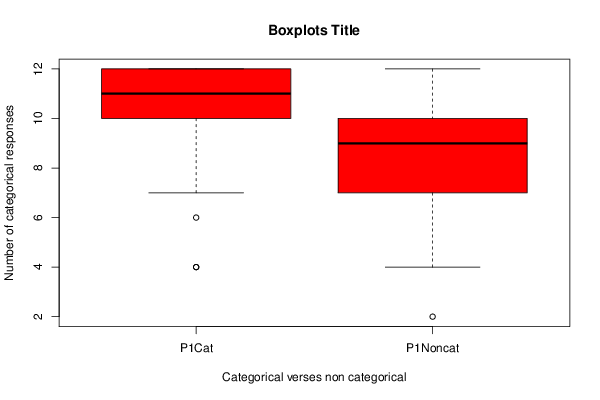## Free Statistics

of Irreproducible Research!

Author's title

# Graph to show verbal interference in relation to the categorical perception...

Author*Unverified author*
R Software Modulerwasp_Tests to Compare Two Means.wasp
Title produced by softwareT-Tests
Date of computationSun, 30 Dec 2012 13:47:01 -0500
Cite this page as followsStatistical Computations at FreeStatistics.org, Office for Research Development and Education, URL https://freestatistics.org/blog/index.php?v=date/2012/Dec/30/t1356893316sglei6pr98yxe6a.htm/, Retrieved Wed, 29 Nov 2023 00:03:13 +0000
Statistical Computations at FreeStatistics.org, Office for Research Development and Education, URL https://freestatistics.org/blog/index.php?pk=204962, Retrieved Wed, 29 Nov 2023 00:03:13 +0000
QR Codes:Original text written by user:
IsPrivate?No (this computation is public)
User-defined keywords
Estimated Impact132
Family? (F = Feedback message, R = changed R code, M = changed R Module, P = changed Parameters, D = changed Data)
-     [Aston University Statistical Software] [Test of Two Means] [2009-11-10 17:16:17] [98fd0e87c3eb04e0cc2efde01dbafab6]
- R P   [Aston University Statistical Software] [Compare Two Means] [2009-11-11 08:09:40] [74be16979710d4c4e7c6647856088456]
-    D    [Aston University Statistical Software] [PY2236 Week 6 dat...] [2009-11-11 12:53:10] [74be16979710d4c4e7c6647856088456]
-   P       [T-Tests] [Week 9 PY2236] [2010-11-24 14:41:08] [74be16979710d4c4e7c6647856088456]
-    D        [T-Tests] [PY2236 Week 9 Dat...] [2010-11-30 18:04:40] [74be16979710d4c4e7c6647856088456]
- RM D          [T-Tests] [PY2236 Week 9 Dat...] [2012-11-27 19:46:15] [74be16979710d4c4e7c6647856088456]
- R  D            [T-Tests] [Graph to show whe...] [2012-12-22 17:09:50] [efeb1429cdbf53ef6e4a9988651e2d36]
- R  D              [T-Tests] [Graph to show cat...] [2012-12-30 18:40:59] [74be16979710d4c4e7c6647856088456]
-    D                  [T-Tests] [Graph to show ver...] [2012-12-30 18:47:01] [d41d8cd98f00b204e9800998ecf8427e] [Current]
Feedback Forum

Post a new message
Dataseries X:
11	7
11	7
11	8
12	6
11	11
10	11
9	5
12	11
9	6
9	8
12	11
9	8
12	10
12	7
9	7
11	11
9	8
11	10
11	7
12	11
11	8
12	11
12	10
12	7
12	10
7	4
9	9
12	10
4	8
10	10
12	9
12	10
6	4
12	6
10	7
12	9
9	10
12	9
9	8
12	10
10	8
12	10
11	5
12	11
12	10
11	9
7	8
12	8
12	6
12	10
10	10
8	9
8	8
10	11
12	9
12	9
12	10
12	12
10	7
12	10
9	5
9	8
12	11
12	10
9	6
11	11
11	9
10	8
10	8
12	4
10	7
9	10
9	6
11	10
12	5
11	12
11	12
12	11
12	8
11	10
12	10
10	9
12	10
4	6
11	8
11	11
12	6
11	2
12	10
9	10

 Summary of computational transaction Raw Input view raw input (R code) Raw Output view raw output of R engine Computing time 1 seconds R Server 'Gwilym Jenkins' @ jenkins.wessa.net

\begin{tabular}{lllllllll}
\hline
Summary of computational transaction \tabularnewline
Raw Input & view raw input (R code)  \tabularnewline
Raw Output & view raw output of R engine  \tabularnewline
Computing time & 1 seconds \tabularnewline
R Server & 'Gwilym Jenkins' @ jenkins.wessa.net \tabularnewline
\hline
\end{tabular}
%Source: https://freestatistics.org/blog/index.php?pk=204962&T=0

[TABLE]
[ROW][C]Summary of computational transaction[/C][/ROW]
[ROW][C]Raw Input[/C][C]view raw input (R code) [/C][/ROW]
[ROW][C]Raw Output[/C][C]view raw output of R engine [/C][/ROW]
[ROW][C]Computing time[/C][C]1 seconds[/C][/ROW]
[ROW][C]R Server[/C][C]'Gwilym Jenkins' @ jenkins.wessa.net[/C][/ROW]
[/TABLE]
Source: https://freestatistics.org/blog/index.php?pk=204962&T=0

Globally Unique Identifier (entire table): ba.freestatistics.org/blog/index.php?pk=204962&T=0

As an alternative you can also use a QR Code:

The GUIDs for individual cells are displayed in the table below:

 Summary of computational transaction Raw Input view raw input (R code) Raw Output view raw output of R engine Computing time 1 seconds R Server 'Gwilym Jenkins' @ jenkins.wessa.net

 Standard Deviations Variable 1 1.734 Variable 2 2.11457

\begin{tabular}{lllllllll}
\hline
Standard Deviations \tabularnewline
Variable 1 & 1.734 \tabularnewline
Variable 2 & 2.11457 \tabularnewline
\hline
\end{tabular}
%Source: https://freestatistics.org/blog/index.php?pk=204962&T=1

[TABLE]
[ROW][C]Standard Deviations[/C][/ROW]
[ROW][C]Variable 1[/C][C]1.734[/C][/ROW]
[ROW][C]Variable 2[/C][C]2.11457[/C][/ROW]
[/TABLE]
Source: https://freestatistics.org/blog/index.php?pk=204962&T=1

Globally Unique Identifier (entire table): ba.freestatistics.org/blog/index.php?pk=204962&T=1

As an alternative you can also use a QR Code:

The GUIDs for individual cells are displayed in the table below:

 Standard Deviations Variable 1 1.734 Variable 2 2.11457PNG link Postscript link PDF link

Parameters (Session):
par1 = two.sided ; par2 = 1 ; par3 = 2 ; par4 = Students T-Test ; par5 = paired ; par6 = 0.0 ; par8 = TRUE ;
Parameters (R input):
par1 = two.sided ; par2 = 1 ; par3 = 2 ; par4 = Students T-Test ; par5 = paired ; par6 = 0.0 ; par7 = ; par8 = TRUE ;
R code (references can be found in the software module):
par8 <- 'TRUE'par7 <- ''par6 <- '0.0'par5 <- 'paired'par4 <- 'Students T-Test'par3 <- '2'par2 <- '1'par1 <- 'two.sided'par2 <- as.numeric(par2)par3 <- as.numeric(par3)par4 <- as.character(par4)par5 <- as.character(par5)par6 <- as.numeric(par6)par7 <- as.numeric(par7)par8 <- as.logical(par8)if ( par5 == 'unpaired') paired <- FALSE else paired <- TRUEx <- t(y)if(par8){bitmap(file='test1.png')(r<-boxplot(x ,xlab=xlab,ylab=ylab,main=main,notch=FALSE,col=2))dev.off()}load(file='createtable')if( par4 == 'Wilcoxon-Mann_Whitney'){a<-table.start()a <- table.row.start(a)a <- table.element(a,'Wilcoxon Test',3,TRUE)a <- table.row.end(a)a <- table.row.start(a)a <- table.element(a,'',1,TRUE)a <- table.element(a,'Statistic',1,TRUE)a <- table.element(a,'P-value',1,TRUE)a <- table.row.end(a)W <- wilcox.test(x[,par2],x[,par3],alternative=par1, paired = paired)a<-table.row.start(a)a<-table.element(a,'Wilcoxon Test',1,TRUE)a<-table.element(a,W$statistic[])a<-table.element(a,round(W$p.value, digits=5) )a<-table.row.end(a)a<-table.end(a)table.save(a,file='mytable.tab')}if( par4 == 'T-Test'){T <- t.test(x[,par2],x[,par3],alternative=par1, paired=paired, mu=par6, conf.level=par7)a<-table.start()a <- table.row.start(a)a <- table.element(a,'T-Test',3,TRUE)a <- table.row.end(a)if(paired){a <- table.row.start(a)a <- table.element(a,'Difference: Mean1 - Mean2',1,TRUE)a<-table.element(a,round(T$estimate, digits=5) )a <- table.row.end(a)}if(!paired){a <- table.row.start(a)a <- table.element(a,'Mean1',1,TRUE)a<-table.element(a,round(T$estimate, digits=5) )a <- table.row.end(a)a <- table.row.start(a)a <- table.element(a,'Mean2',1,TRUE)a<-table.element(a,round(T$estimate, digits=5) )a <- table.row.end(a)}a <- table.row.start(a)a <- table.element(a,'T Statistic',1,TRUE)a<-table.element(a,round(T$statistic, digits=5) )a <- table.row.end(a)a <- table.row.start(a)a <- table.element(a,'P-value',1,TRUE)a<-table.element(a,round(T$p.value, digits=5) )a <- table.row.end(a)a <- table.row.start(a)a <- table.element(a,'Lower Confidence Limit',1,TRUE)a<-table.element(a,round(T$conf.int, digits=5) )a <- table.row.end(a)a<-table.row.start(a)a <- table.element(a,'Upper Confidence Limit',1,TRUE)a<-table.element(a,round(T\$conf.int, digits=5) )a <- table.row.end(a)a<-table.end(a)table.save(a,file='mytable.tab')}a<-table.start()a <- table.row.start(a)a <- table.element(a,'Standard Deviations',3,TRUE)a <- table.row.end(a)a <- table.row.start(a)a <- table.element(a,'Variable 1',1,TRUE)a<-table.element(a,round(sd(x[,par2], na.rm=TRUE), digits=5) )a <- table.row.end(a)a <- table.row.start(a)a <- table.element(a,'Variable 2',1,TRUE)a<-table.element(a,round(sd(x[,par3], na.rm=TRUE), digits=5) )a <- table.row.end(a)a<-table.end(a)table.save(a,file='mytable1.tab')